# Duncan Lardeau Juvenile Rainbow Trout Abundance 2018

The suggested citation for this analytic report is:

Thorley, J.L. and Dalgarno, S. (2018) Duncan Lardeau Juvenile Rainbow Trout Abundance 2018. A Poisson Consulting Analysis Report. URL: https://www.poissonconsulting.ca/f/679886444.

## Background

Rainbow Trout rear in the Lardeau and Lower Duncan rivers. Since 2006 (with the exception of 2015) annual spring snorkel surveys have been conducted to estimate the abundance and distribution of age-1 Rainbow Trout. From 2006 to 2010 the surveys were conducted at fixed index sites. Since 2011 fish observations have been mapped to the river based on their spatial coordinates as recorded by GPS.

The primary aims of the current analyses were to:

1. Estimate the spring abundance of age-1 fish by year.
2. Estimate the egg deposition.
3. Estimate the stock-recruitment relationship between the egg deposition and the abundance of age-1 recruits the following spring.
4. Estimate a Limit Reference Point (LRP) for the egg deposition (and number of spawners).
5. Estimate the maximum reproductive rate (spawners per spawner at low density).
6. Rerun preliminary yield-per-recruit estimates based on the latest results.

## Methods

### Data Preparation

The data were provided by the Ministry of Forests, Lands and Natural Resource Operations (MFLNRO). The historical and current snorkel count data were manipulated using R version 3.5.1 (R Core Team 2017) and organised in an SQLite database.

### Data Analysis

Hierarchical Bayesian models were fitted to the data using R version 3.5.1 (R Core Team 2017), Stan 2.16.0 (Carpenter et al. 2017) and JAGS 4.2.0 (Plummer 2015) and the mbr family of packages.

Unless indicated otherwise, the models used prior distributions that were vague in the sense that they did not affect the posterior distributions (Kery and Schaub 2011, 36). The posterior distributions were estimated from 2,000 Markov Chain Monte Carlo (MCMC) samples thinned from the second halves of three chains (Kery and Schaub 2011, 38–40). Model convergence was confirmed by ensuring that $$\hat{R} < 1.1$$ (Kery and Schaub 2011, 40) for each of the monitored parameters in the model (Kery and Schaub 2011, 61). Where relevant, model adequacy was confirmed by examination of residual plots.

The posterior distributions of the fixed (Kery and Schaub 2011, 75) parameters are summarised in terms of the point estimate, standard deviation (sd), the z-score, lower and upper 95% confidence/credible limits (CLs) and the p-value (Kery and Schaub 2011, 37, 42). The estimate is the median (50th percentile) of the MCMC samples, the z-score is $$\mathrm{mean}/\mathrm{sd}$$ and the 95% CLs are the 2.5th and 97.5th percentiles. A p-value of 0.05 indicates that the lower or upper 95% CL is 0.

Variable selection was achieved by dropping fixed (Kery and Schaub 2011, 77–82) variables with two-sided p-values $$\geq$$ 0.05 (Kery and Schaub 2011, 37, 42) and random variables with percent relative errors $$\geq$$ 80%.

The results are displayed graphically by plotting the modeled relationships between particular variables and the response with 80% or 95% credible intervals (CIs) with the remaining variables held constant. In general, continuous and discrete fixed variables are held constant at their mean and first level values respectively while random variables are held constant at their typical values (expected values of the underlying hyperdistributions) (Kery and Schaub 2011, 77–82). Where informative the influence of particular variables is expressed in terms of the effect size (i.e., percent change in the response variable) with 95% CIs (Bradford, Korman, and Higgins 2005).

### Model Descriptions

#### Length Correction

The annual bias (inaccuracy) and error (imprecision) in observer’s fish length estimates when spotlighting (standing) and snorkeling were quantified from the divergence of their length distribution from the length distribution for all observers (including measured fish) in that year. More specifically, the length correction that minimised the Jensen-Shannon divergence (Lin 1991) between the two distributions provided a measure of the inaccuracy while the minimum divergence (the Jensen-Shannon divergence was calculated with log to base 2 which means it lies between 0 and 1) provided a measure of the imprecision.

After correcting the fish lengths, age-1 individuals were assumed to be those with a fork length $$\leq$$ 100 mm.

#### Abundance

The abundance was estimated from the count data using an overdispersed Poisson model (Kery and Schaub 2011, 55–56). The annual abundance estimates represent the total number of fish in the study area.

Key assumptions of the abundance model include:

• The lineal fish density varies with year, useable width and river kilometer as a polynomial, and randomly with site.
• The observer efficiency at marking sites varies by study design (GPS versus Index).
• The observer efficiency also varies by visit type (marking versus count) within study design and randomly by snorkeller.
• The expected count at a site is the expected lineal density multiplied by the site length, the observer efficiency and the proportion of the site surveyed.
• The residual variation in the actual count is gamma-Poisson distributed.

#### Condition

The condition of fish with a fork length $$\geq$$ 500 mm was estimated via an analysis of mass-length relations (He et al. 2008).

More specifically the model was based on the allometric relationship

$W = \alpha_c L^{\beta_c}$

where $$W$$ is the weight (mass), $$\alpha_c$$ is the coefficent, $$\beta_c$$ is the exponent and $$L$$ is the length.

To improve chain mixing the relation was log-transformed, i.e.,

$\log(W) = \log(\alpha_c) + \beta_c \log(L).$

Key assumptions of the condition model include:

• $$\alpha_c$$ can vary randomly by year.
• The residual variation in weight is log-normally distributed.

#### Fecundity

The fecundity of females with a fork length $$\geq$$ 500 mm was estimated via an analysis of fecundity-mass relations.

More specifically the model was based on the allometric relationship

$F = \alpha_f W^{\beta_f}$

where $$F$$ is the fecundity, $$\alpha_f$$ is the coefficent, $$\beta_f$$ is the exponent and $$W$$ is the weight.

To improve chain mixing the relation was log-transformed.

Key assumptions of the fecundity model include:

• The residual variation in fecundity is log-normally distributed.

#### Spawner Size

The average length of the spawners in each year (for years for which it was unavailable) was estimated from the mean weight of Rainbow Trout in the Kootenay Lake Rainbow Trout Mailout Survey (KLRT) using a linear regression. This approach was suggested by Rob Bison.

#### Egg Deposition

The egg deposition in each year was estimated by

1. converting the average length of spawners to the average weight using the condition relationship for a typical year
2. adjusting the average weight by the annual condition effect (interpolating where unavailable)
3. converting the average weight to the average fecundity using the fecundity relationship
4. multiplying the average fecundity by the AUC based estimate of the number of females (assuming a sex ratio of 1:1)

#### Stock-Recruitment

The relationship between the number of eggs ($$E$$) and the abundance of age-1 individuals the following spring ($$R$$) was estimated using a Beverton-Holt stock-recruitment model (Walters and Martell 2004):

$R = \frac{\alpha_s \cdot E}{1 + \beta_s \cdot E} \quad,$

where $$\alpha_s$$ is the maximum number of recruits per egg (egg survival), and $$\beta_s$$ is the density dependence.

Key assumptions of the stock-recruitment model include:

• The residual variation in the number of recruits is log-normally distributed with the standard deviation scaling with the uncertainty in the number of recruits.

The age-1 carrying capacity ($$K$$) is given by:

$K = \frac{\alpha_s}{\beta_s} \quad.$

and the $$E_{K/2}$$ Limit Reference Point (Mace 1994, E_{0.5 R_{max}}), which corresponds to the stock (number of eggs) that produce 50% of the maximum recruitment ($$K$$), by $E_{K/2} = \frac{1}{\beta_s}$

The LRP was also converted into a number of spawners in a typical year (assuming 6,000 eggs per spawner and a sex ratio of 1:1).

#### Environmental

The mean daily discharge in the Lardeau at the Water Survey of Canada Marblehead site (08NH007) was plotted during the emergence period to see if an unusual hydrograph may have been responsible for the high recruitment from the 2005 spawn year.

#### Reproductive Rate

The maximum reproductive rate (the number of spawners per spawner at low density) not accounting for fishing mortality was calculated by multiplying $$\beta_s$$ (number of recruits per egg at low density) from the stock-recruitment relationship by the inlake survival by the average number of eggs per spawner in a typical year (assumed to be 3,000 based on 6,000 eggs per spawner and a sex ratio of 1:1). The inlake survival from age-1 to spawning was calculated by dividing the subsequent number of spawners by the number of recruits assuming that equal numbers of fish spawn at age 5, 6 and 7.

#### Yield-per-Recruit

The optimal capture rate and egg deposition was estimated using the ypr R package.

### Abundance

  data {

int<lower=0> nMarked;
int<lower=0> Marked[nMarked];
int<lower=0> Resighted[nMarked];
int<lower=0> IndexMarked[nMarked];

int<lower=0> nObs;
int Marking[nObs];
int Index[nObs];
int<lower=0> nSwimmer;
int<lower=0> Swimmer[nObs];
int<lower=0> nYear;
int<lower=0> Year[nObs];
real Width[nObs];
real Rkm[nObs];
int<lower=0> nSite;
int<lower=0> Site[nObs];

real SiteLength[nObs];
real SurveyProportion[nObs];

int Count[nObs];
}
parameters {
real bEfficiency;
real bEfficiencyIndex;

real bDensity;
vector[nYear] bDensityYear;
real bDensityWidth;
vector bDensityRkm;
real<lower=0> sDensitySite;
vector[nSite] bDensitySite;

real bEfficiencyMarking;
real bEfficiencyMarkingIndex;

real<lower=0,upper=5> sEfficiencySwimmer;
vector[nSwimmer] bEfficiencySwimmer;

real<lower=0> sDispersion;
}
model {

vector[nObs] eDensity;
vector[nObs] eEfficiency;
vector[nObs] eAbundance;
vector[nObs] eCount;

sDispersion ~ gamma(0.01, 0.01);

bDensity ~ normal(0, 2);
bDensityRkm ~ normal(0, 2);
sDensitySite ~ uniform(0, 5);
bDensitySite ~ normal(0, sDensitySite);
bDensityWidth ~ normal(0, 2);
bDensityYear ~ normal(0, 5);

bEfficiency ~ normal(0, 5);
bEfficiencyIndex ~ normal(0, 5);
bEfficiencyMarking ~ normal(0, 5);
bEfficiencyMarkingIndex ~ normal(0, 5);
sEfficiencySwimmer ~ uniform(0, 5);
bEfficiencySwimmer ~ normal(0, sEfficiencySwimmer);

for (i in 1:nMarked) {
target += binomial_lpmf(Resighted[i] | Marked[i],
inv_logit(
bEfficiency +
bEfficiencyIndex * IndexMarked[i] +
bEfficiencyMarking +
bEfficiencyMarkingIndex * IndexMarked[i]
));
}

for (i in 1:nObs) {
eDensity[i] = exp(bDensity +
bDensityRkm * Rkm[i] +
bDensityRkm * pow(Rkm[i], 2.0) +
bDensityRkm * pow(Rkm[i], 3.0) +
bDensityRkm * pow(Rkm[i], 4.0) +
bDensitySite[Site[i]] +
bDensityYear[Year[i]] +
bDensityWidth * log(Width[i]));

eEfficiency[i] = inv_logit(
bEfficiency +
bEfficiencyIndex * Index[i] +
bEfficiencyMarking * Marking[i] +
bEfficiencyMarkingIndex * Index[i] * Marking[i] +
bEfficiencySwimmer[Swimmer[i]]);

eAbundance[i] = eDensity[i] * SiteLength[i];

eCount[i] = eAbundance[i] * eEfficiency[i] * SurveyProportion[i];
}

target += neg_binomial_2_lpmf(Count | eCount, sDispersion);
}

Template 1. Abundance model description.

### Condition

 data {
int nYear;
int nObs;

vector[nObs] Length;
vector[nObs] Weight;
int Year[nObs];

parameters {
real bWeight;
real bWeightLength;
real sWeightYear;

vector[nYear] bWeightYear;
real sWeight;

model {

vector[nObs] eWeight;

bWeight ~ normal(-10, 5);
bWeightLength ~ normal(3, 2);

sWeightYear ~ normal(-2, 5);

for (i in 1:nYear) {
bWeightYear[i] ~ normal(0, exp(sWeightYear));
}

sWeight ~ normal(-2, 5);
for(i in 1:nObs) {
eWeight[i] = bWeight + bWeightLength * log(Length[i]) + bWeightYear[Year[i]];
Weight[i] ~ lognormal(eWeight[i], exp(sWeight));
}
..

Template 2.

### Fecundity

 data {
int nObs;

vector[nObs] Weight;
vector[nObs] Fecundity;

parameters {
real bFecundity;
real bFecundityWeight;
real sFecundity;

model {

vector[nObs] eFecundity;

bFecundity ~ uniform(0, 5);
bFecundityWeight ~ uniform(0, 2);

sFecundity ~ uniform(0, 1);
for(i in 1:nObs) {
eFecundity[i] = log(bFecundity) + bFecundityWeight * log(Weight[i]);
Fecundity[i] ~ lognormal(eFecundity[i], sFecundity);
}
..

Template 3.

### Stock-Recruitment

model {
a ~ dunif(0, 1)
b ~ dunif(0, 0.1)
sScaling ~ dunif(0, 5)

eRecruits <- a * Stock / (1 + Stock * b)

for(i in 1:nObs) {
esRecruits[i] <- SDLogRecruits[i] * sScaling
Recruits[i] ~ dlnorm(log(eRecruits[i]), esRecruits[i]^-2)
}
..

Template 4. Stock-Recruitment model description.

## Results

### Abundance

Table 1. Parameter descriptions.

Parameter Description
bDensity Intercept for log(eDensity)
bDensityRkm[x] xth-order polynomial coefficients of effect of river kilometer on bDensity
bDensitySite[i] Effect of ith Site on bDensity
bDensityWidth Effect of Width on bDensity
bDensityYear[i] Effect of ith Year on bDensity
bEfficiency Intercept of logit(eEfficiency)
bEfficiencyIndex Effect of Index on bEfficiency
bEfficiencyMarking Effect of Marking on bEfficiency
bEfficiencyMarkingIndex Effect of Marking and Index on bEfficiency
bEfficiencySwimmer[i] Effect of ith Swimmer on bEfficiency
eAbundance[i] Expected abundance of fish at site of ith visit
eCount[i] Expected total number of fish at site of ith visit
eDensity[i] Expected lineal density of fish at site of ith visit
eEfficiency[i] Expected observer efficiency on ith visit
Index Whether the ith visit was to an index site
Marking[i] Whether the ith visit was to a site with marked fish
Rkm[i] River kilometer of ith visit
sDensitySite SD of bDensitySite
sDispersion Overdispersion of Count[i]
sEfficiencySwimmer SD of bEfficiencySwimmer
Site[i] Site of ith visit
SiteLength[i] Length of site of ith visit
SurveyProportion[i] Proportion of site surveyed on ith visit
Swimmer[i] Snorkeler on ith site visit
Width[i] Useable width of site on ith visit
Year[i] Year of ith site visit

Table 2. Model coefficients.

term estimate sd zscore lower upper pvalue
bDensity -0.6963658 1.1641306 -0.5837528 -2.8674006 1.6429677 0.5733
bDensityRkm -0.2042877 0.0944545 -2.1937619 -0.3948469 -0.0329674 0.0267
bDensityRkm 0.6567185 0.1097909 5.9826906 0.4389913 0.8767718 0.0007
bDensityRkm 0.0391887 0.0378328 1.0529303 -0.0309667 0.1144436 0.2853
bDensityRkm -0.2992126 0.0360876 -8.2945201 -0.3710949 -0.2305983 0.0007
bDensityWidth 0.0883220 0.0273180 3.2481475 0.0361958 0.1421477 0.0027
bDensityYear 0.7529302 1.2132431 0.6149444 -1.6731131 2.9758967 0.5253
bDensityYear -0.1643764 1.2059441 -0.1412118 -2.6009230 2.1341145 0.9080
bDensityYear -0.4624095 1.1997102 -0.4098222 -2.8896459 1.7299649 0.6920
bDensityYear -0.4846544 1.2095951 -0.4331476 -2.9586656 1.7840561 0.6813
bDensityYear -0.1407823 1.2185438 -0.1595103 -2.6134068 2.1441766 0.9000
bDensityYear 0.2360800 1.1643191 0.2112010 -2.0994908 2.4362783 0.8507
bDensityYear 0.2463119 1.1625706 0.2259988 -2.1796061 2.3901774 0.8320
bDensityYear -0.0972376 1.1653885 -0.0839833 -2.4758856 2.0959280 0.9387
bDensityYear -0.4997545 1.1614718 -0.4126908 -2.8641777 1.6887987 0.7080
bDensityYear -1.4189572 1.1619014 -1.2142423 -3.8173203 0.7722614 0.2253
bDensityYear -0.7864326 1.1651559 -0.6748531 -3.1518673 1.3770294 0.5040
bDensityYear -0.2520908 1.1673984 -0.2223446 -2.6428958 1.8999666 0.8493
bEfficiency -1.7735459 0.1555540 -11.3621426 -2.0770923 -1.4639251 0.0007
bEfficiencyIndex 0.1989810 0.4262533 0.4846959 -0.6190535 1.0451957 0.6347
bEfficiencyMarking 0.1654221 0.1076543 1.5317465 -0.0390752 0.3708288 0.1267
bEfficiencyMarkingIndex 1.6046481 0.4097403 3.9097275 0.8008554 2.3872085 0.0013
sDensitySite 0.6320314 0.0381650 16.5604943 0.5599186 0.7107649 0.0007
sDispersion 1.2761934 0.0677495 18.8599429 1.1517084 1.4189128 0.0007
sEfficiencySwimmer 0.4005182 0.1446675 2.9705127 0.2279183 0.7822507 0.0007

Table 3. Model summary.

n K nchains niters nthin ess rhat converged
3015 25 3 500 2 256 1.012 TRUE

Table 4. Summary survey information by year and river.

Year River Sites SurveyLength SurveyPercent Fish Marked Resighted
2006 Duncan 0 0.0 0 0 0 0
2006 Lardeau 34 2.0 1 620 36 22
2007 Duncan 0 0.0 0 0 0 0
2007 Lardeau 47 2.7 2 260 0 0
2008 Duncan 0 0.0 0 0 0 0
2008 Lardeau 97 7.5 5 618 102 54
2009 Duncan 0 0.0 0 0 0 0
2009 Lardeau 83 5.0 4 390 0 0
2010 Duncan 0 0.0 0 0 0 0
2010 Lardeau 48 2.5 2 303 0 0
2011 Duncan 32 1.8 4 149 0 0
2011 Lardeau 264 15.8 11 1975 0 0
2012 Duncan 71 3.8 7 516 0 0
2012 Lardeau 257 15.2 11 1694 43 6
2013 Duncan 117 7.1 14 840 62 11
2013 Lardeau 308 18.0 13 1237 155 29
2014 Duncan 77 4.9 9 282 72 16
2014 Lardeau 273 16.3 12 1005 100 15
2016 Duncan 70 3.6 7 137 0 0
2016 Lardeau 257 14.7 10 321 16 3
2017 Duncan 92 5.5 11 255 30 5
2017 Lardeau 510 30.1 21 1370 29 0
2018 Duncan 19 1.0 2 34 0 0
2018 Lardeau 197 10.8 8 899 93 15

Table 5. Summary survey information by year.

Year Sites SurveyLength SurveyPercent Fish Marked Resighted
2006 34 2.0 1 620 36 22
2007 47 2.7 1 260 0 0
2008 97 7.5 4 618 102 54
2009 83 5.0 3 390 0 0
2010 48 2.5 1 303 0 0
2011 296 17.6 9 2124 0 0
2012 328 19.0 10 2210 43 6
2013 425 25.1 13 2077 217 40
2014 350 21.1 11 1287 172 31
2016 327 18.3 10 458 16 3
2017 602 35.6 19 1625 59 5
2018 216 11.8 6 933 93 15

### Condition

Table 6. Parameter descriptions.

Parameter Description
bWeight Intercept of log(eWeight)
bWeightLength Intercept of effect of log(Length) on bWeight
bWeightYear[i] Effect of ith Year on bWeight
eWeight[i] Expected Weight of ith fish
Length[i] Fork length of ith fish
sWeight Log standard deviation of residual variation in log(Weight)
sWeightYear Log standard deviation of bWeightYear
Weight[i] Recorded weight of ith fish
Year[i] Year ith fish was captured

Table 7. Model coefficients.

term estimate sd zscore lower upper pvalue
bWeight -12.636773 0.2221935 -56.88024 -13.083567 -12.207840 7e-04
bWeightLength 3.201633 0.0334451 95.73530 3.137294 3.268442 7e-04
sWeight -1.906158 0.0213756 -89.16001 -1.946579 -1.861919 7e-04
sWeightYear -2.124485 0.1644743 -12.86048 -2.426690 -1.781469 7e-04

Table 8. Model summary.

n K nchains niters nthin ess rhat converged
1113 4 3 500 2 350 1.007 TRUE

### Fecundity

Table 9. Parameter descriptions.

Parameter Description
bFecundity Intercept of eFecundity
bFecundityWeight Effect of log(Weight) on log(bFecundity)
eFecundity[i] Expected Fecundity of ith fish
Fecundity[i] Fecundity of ith fish (eggs)
sFecundity SD of residual variation in log(Fecundity)
Weight[i] Weight of ith fish (mm)

Table 10. Model coefficients.

term estimate sd zscore lower upper pvalue
bFecundity 3.9209077 0.9120282 4.098084 1.6821483 4.9494551 7e-04
bFecundityWeight 0.8598367 0.0328506 26.456045 0.8320117 0.9545648 7e-04
sFecundity 0.1272536 0.0206999 6.256100 0.0956913 0.1762694 7e-04

Table 11. Model summary.

n K nchains niters nthin ess rhat converged
22 3 3 500 2 248 1.007 TRUE

### Egg Deposition

Table 12. The estimate total egg deposition by spawn year.

Year Length Condition Weight Fecundity Spawners Eggs
2000 780.1010 1.0393491 6139.137 7060.404 593.3613 2094685.3
2001 714.3372 1.0549815 4699.355 5594.193 316.1345 884258.6
2002 686.9467 1.0706139 4206.923 5082.432 398.9916 1013923.8
2003 712.0871 1.0862463 4789.665 5688.156 501.1765 1425384.9
2004 720.0000 1.0967083 5010.301 5916.112 628.4034 1858852.5
2005 770.0000 1.0387250 5884.646 6803.861 608.5714 2070317.8
2006 830.0000 0.9674553 6967.814 7881.746 652.9412 2573158.3
2007 739.8839 0.9783361 4876.891 5778.040 691.6807 1998279.2
2008 678.6507 0.9892170 3738.972 4588.655 514.0336 1179361.4
2009 669.5240 1.0363650 3751.517 4602.047 1058.7395 2436184.5
2010 730.0000 0.9693155 4628.480 5520.909 1251.5126 3454743.5
2011 810.8447 1.0463752 6994.031 7907.960 1432.5210 5664159.6
2012 854.1830 0.9616737 7594.224 8493.610 1532.0168 6506177.0
2013 828.6478 0.8769723 6283.148 7204.020 1250.9244 4505842.4
2014 780.0000 0.7922708 4677.790 5571.812 932.0168 2596511.3
2015 561.1913 0.7720349 1589.231 2202.325 301.2605 331736.8
2016 580.0000 0.7517990 1719.790 2356.435 162.1849 191089.0
2017 530.0000 0.8111756 1390.503 1963.320 256.2185 251519.4

### Stock-Recruitment

Table 13. Parameter descriptions.

Parameter Description
a Recruits per Stock at low density
b Density-dependence
eRecruits[i] Expected number of recruits from ith spawn year
esRecruits[i] Expected SD of residual variation in Recruits
Recruits[i] Number of recruits from ith spawn year
SDLogRecruits[i] Standard deviation of uncertainty in log(Recruits[i])
sScaling Scaling term for SD of residual variation in log(eRecruits)
Stock[i] Number of egg in ith spawn year

#### All

Table 14. Model coefficients.

term estimate sd zscore lower upper pvalue
a 0.6480776 0.2024845 3.199622 0.2624457 0.9850739 7e-04
b 0.0000057 0.0000024 2.435438 0.0000018 0.0000109 7e-04
sScaling 2.2045744 0.5784344 4.003556 1.4971373 3.7251551 7e-04

Table 15. Model summary.

n K nchains niters nthin ess rhat converged
12 3 3 500 100 1296 1.001 TRUE

Table 16. Estimated carry capacity (with 95% CRIs).

estimate lower upper
115000 77900 173000

Table 17. Estimated reference points (with 80% CRIs).

Metric estimate lower upper
eggs 176000.00000 111000 356000.0000
spawners 58.66667 37 118.6667

#### Reduced

Table 18. Model coefficients.

term estimate sd zscore lower upper pvalue
a 0.6821462 0.2008683 3.325851 0.288619 0.9829678 7e-04
b 0.0000061 0.0000025 2.517096 0.000002 0.0000113 7e-04
sScaling 2.1725201 0.5938579 3.854503 1.453242 3.8570925 7e-04

Table 19. Model summary.

n K nchains niters nthin ess rhat converged
11 3 3 500 100 1356 1.001 TRUE

Table 20. Estimated carry capacity (with 95% CRIs).

estimate lower upper
111000 76100 165000

Table 21. Estimated reference points (with 80% CRIs).

Metric estimate lower upper
eggs 165000 106000.00000 326000.0000
spawners 55 35.33333 108.6667

### Abundance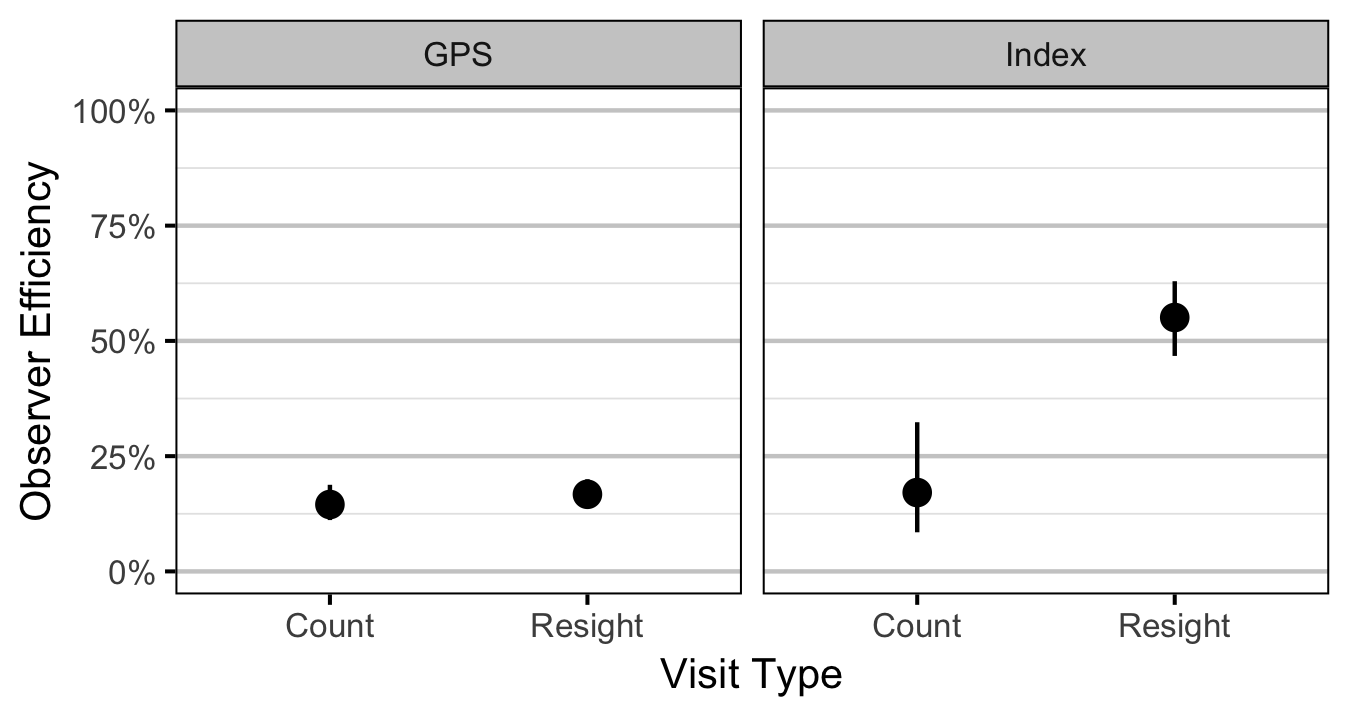Figure 2. Predicted observer efficiency for age-1 Rainbow Trout by visit type and study design (with 95% CRIs).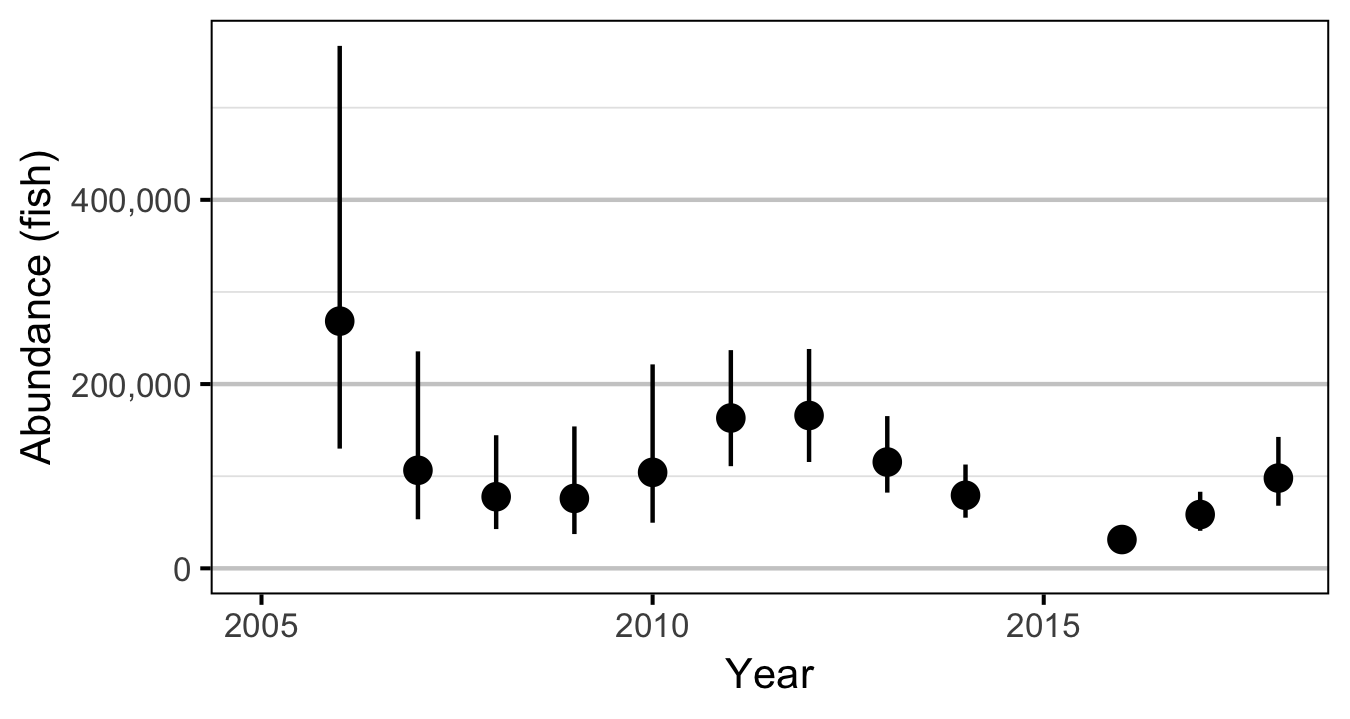Figure 3. Predicted abundance of age-1 Rainbow Trout in the Duncan and Lardeau Rivers by year (with 95% CRIs).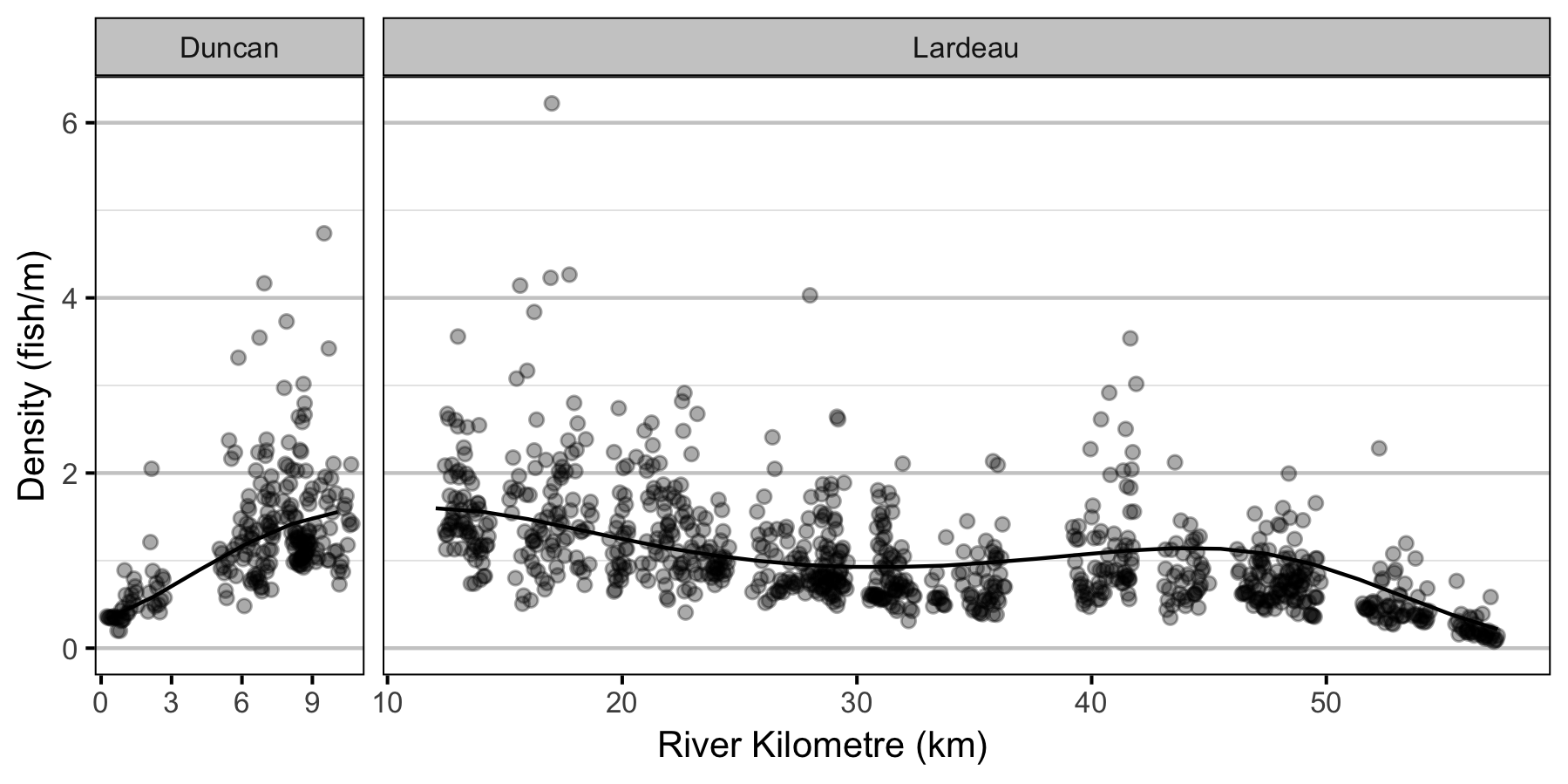Figure 4. Predicted lineal density of age-1 Rainbow Trout in 2010 by river kilometre (with 95% CRIs).

### Condition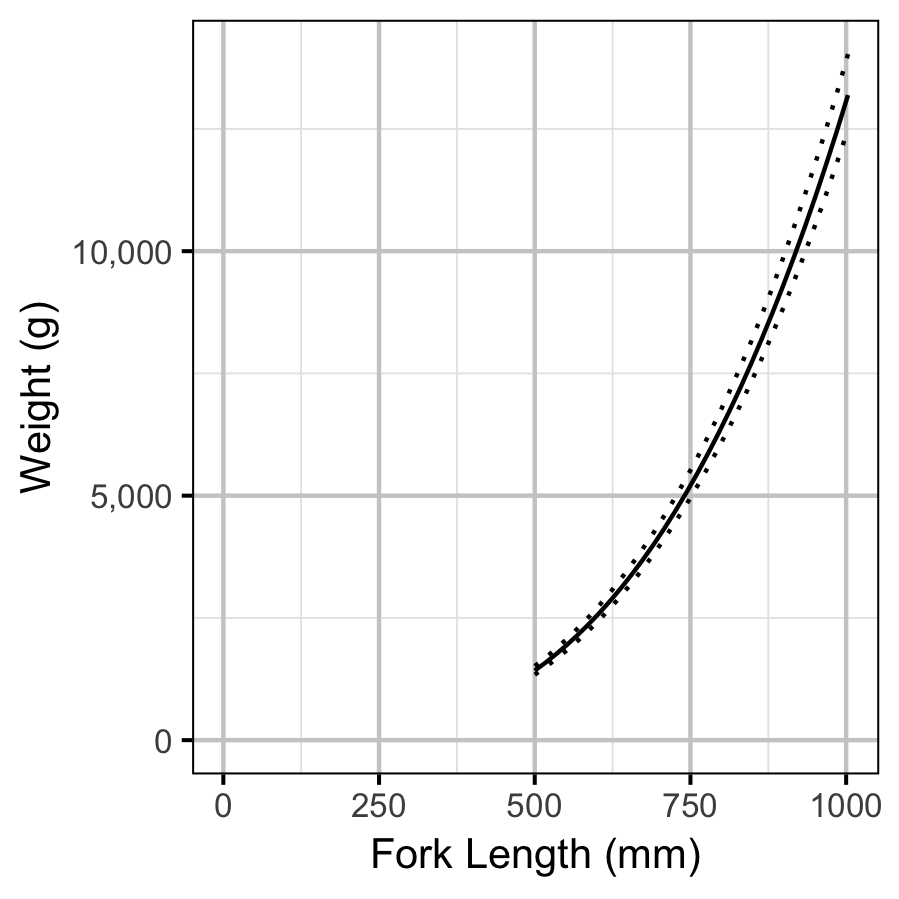Figure 5. The weight-length relationship (with 95% CRIs).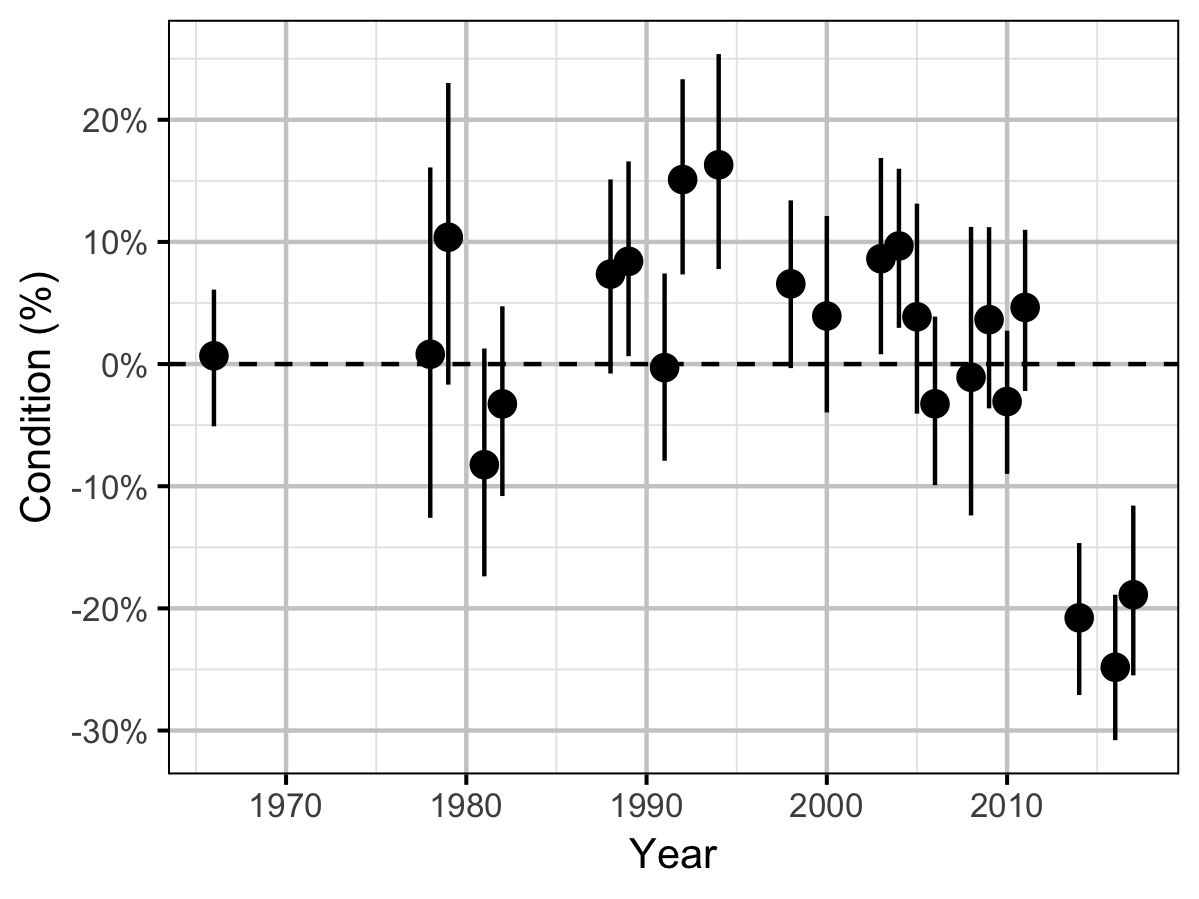Figure 6. The percent change in the body condition for an average length fish relative to a typical year by year (with 95% CRIs).

### Spawner Size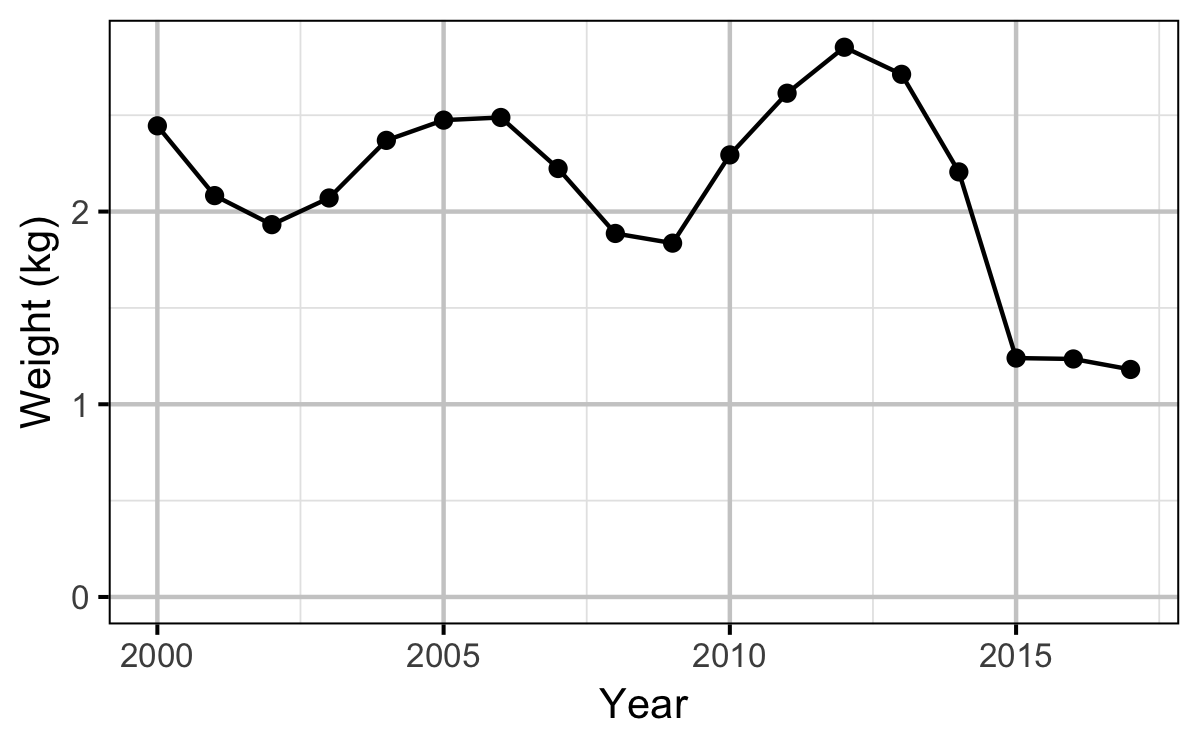Figure 8. The mean weight of Rainbow Trout in the KLRT by year.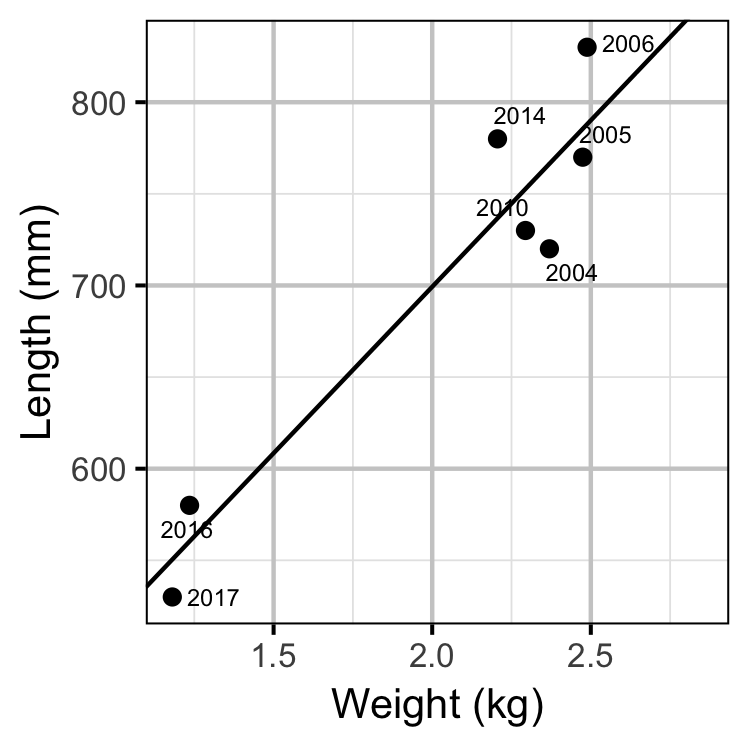Figure 9. The mean length of spawning Rainbow Trout by the mean weight of Rainbow Trout in the KLRT.

### Stock-Recruitment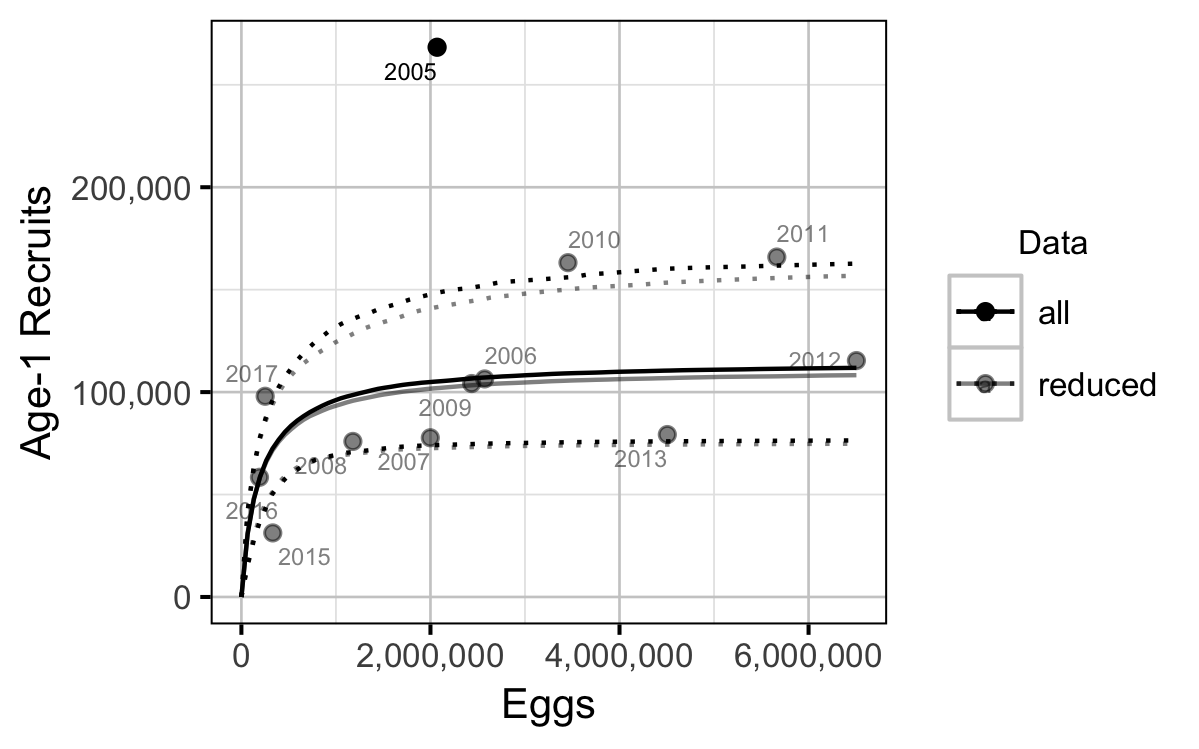Figure 13. Predicted stock-recruitment relationship between egg deposition and age-1 recruits by data set (with 95% CRIs). The labels indicate the spawn year.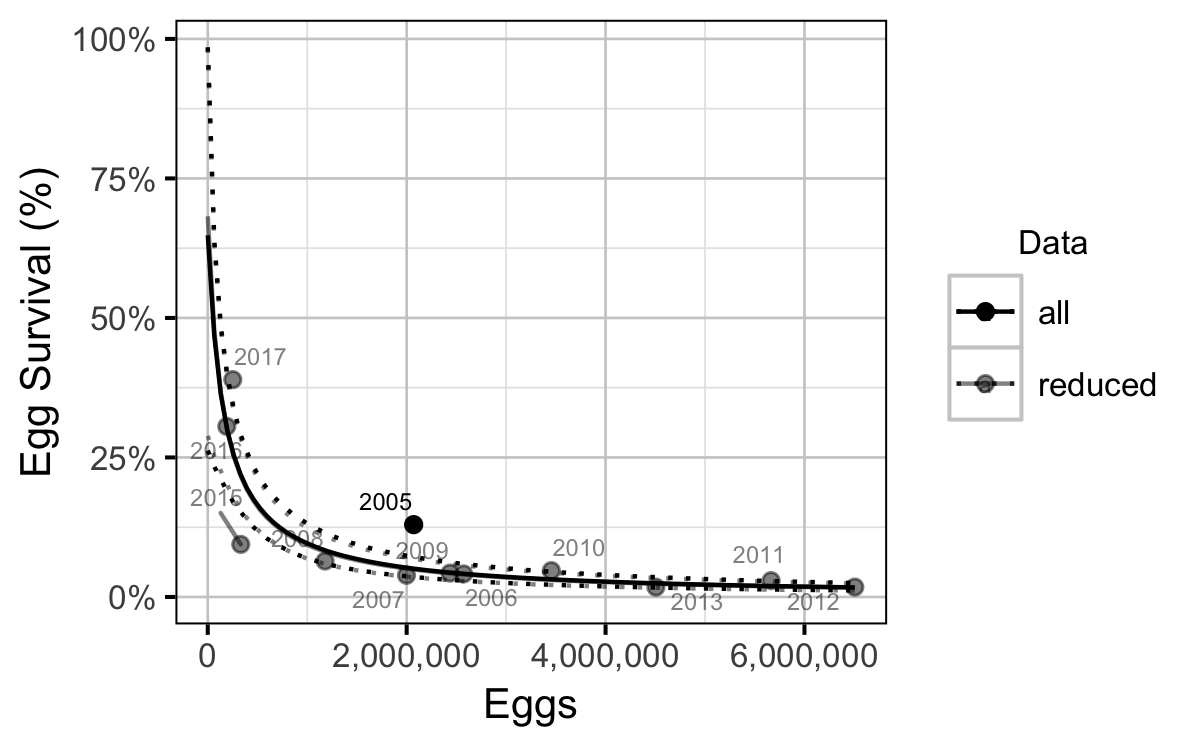Figure 14. Predicted egg survival by egg deposition by data set (with 95% CRIs). The labels indicate the spawn year.

### Environmental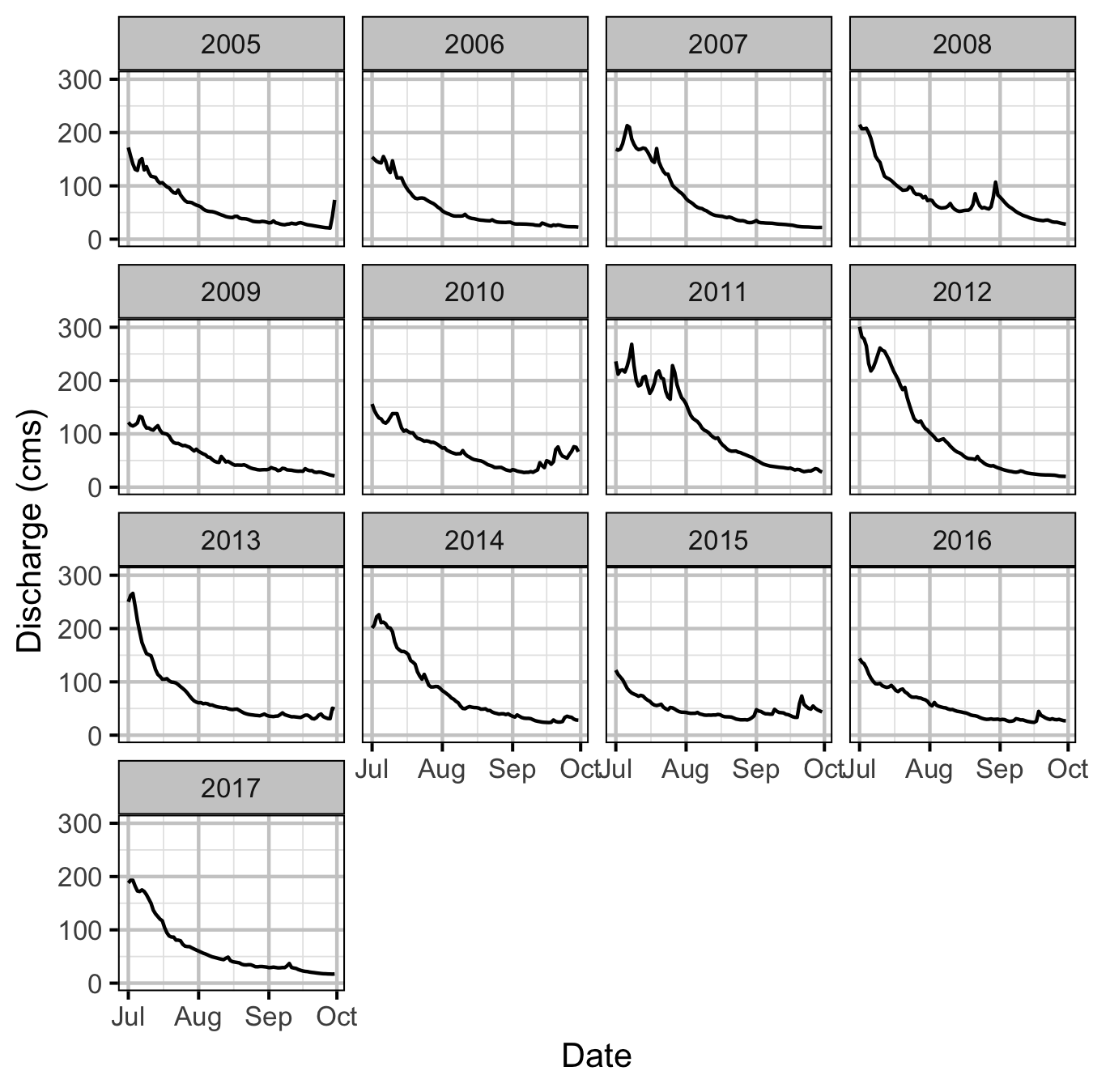Figure 15. Mean daily discharge in the Lardeau River at Marblehead during emergence by spawn year.

### Reproductive Rate

#### Conclusions

• The Limit Reference Point is 356,000 eggs or 118 AUC spawners (based on fecundity in a typical year).
• The egg deposition has fallen below the LRP since 2015.
• Exclusion of the large recruitment estimate from the 2005 spawn year has little effect on the estimates of the LRP.
• The maximum reproductive rate increased from around 12.5 in the early to mid 2000s to over 30 in 2007 before dropping to under 5 in 2010. The changes reflect shifts in the inlake survival.

### Recommendations

• Develop a Limit Reference Point that ensures a effective population size ($$N_e$$) of 500.
• Develop an Upper Reference Point that ensures adequate Kokanee recruitment.

## Acknowledgements

The organisations and individuals whose contributions have made this analysis report possible include: